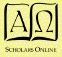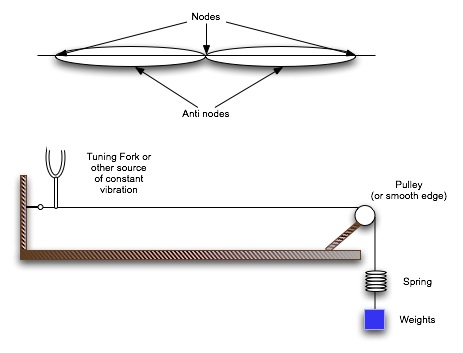Physics Core/AP 1 and 2

#### Course Materials are always under revision! Weblecture content may change anytime prior to two weeks before scheduled chat session for content.Standing Wavies

# Lab: Standing Waves

#### Materials

• Tuning fork by preference, regular 2-prong cooking fork if necessary. More than one at different pitches if at all possible.
• Nylon thread or fishing line.
• Pulley or glass cup.
• Light-weight container.
• Weights (nickels = 5 gm, aspirin tables or other uniform pill -- use label on bottle.

#### Procedure

1. Chose a "light weight container" that you can tie to one end of the thread. It should be small enough to swing freely in your setup, but with an open top so that you can add weights while the thread is vibrating and observe the change in pitch.
2. Place your pulley at the edge of your setup table. It should be large enough to allow the thread to drop free of interference from the table. If you don't have a pulley, tape a glass jar to the edge of your setup table so that it juts out and a thread passed over it will clear the table.
3. Secure the base of the tuning fork so that it can vibrate but also support a small pull in the horizontal direction without falling over.
4. Tie one end of the thread to the fork and the other to your "light weight container".
5. Pass the thread over your pulley.
6. Your setup should look like the diagram below:7. Describe your tuning fork. If you use an actual tuning fork, record its frequency. If you use another fork, attempt to guess its pitch by comparing to to a piano or other source of single-tone musical notes.
8. Set the tuning fork vibrating.
9. Add weights to the container until you get a standing wave of one or more loops in the string. Count the loops and record the weight.
10. Continue adding weights and recording weight and number of standing waves for at least three standing wave situations.
11. Repeat if possible for more than one frequency (if you have multiple sources for sustained tones) and/or for different threads.

#### Data Handling

1. Create a table of raw data showing mass or weight, length L between vibration source and pulley, and number of nodes observed. (see diagram)
2. Calculate the wavelength associated with each node.
3. Study the formula for velocity in a string, v = sqrt [ Force/ (mass/length)].
• M = suspended mass
• g = gravitational acceleration = 9.8 m/sec2
• m = mass of string and L = length of string, so m/L = the linear density of the string μ.
• Show that v = sqrt(F/μ) leads to the relationship frequency = n/2L * sqrt (F/μ).
4. Using the frequency equation, and your observations of wavelength, frequency [from the tuning fork or other sustained tone generator), n, L, M (so that F = Mg), calculate μ for each trial.
5. Average your results and discuss sources of error.

#### Report

1. Describe your materials, equipment, an dprocedures in sufficient detail that your fellow students could repeat your experiment.
2. Report your data. Be sure to indicate the amount of error in your measurements. For example, if you can only measure a mass of 25 gms within 1 grm, your error would be 25 ± 1, or 1/25 = 4%.
3. Present your data in an organized form, preferably in a table, in such a way it is easy to compare results as you repeate trials or vary a specific contributing factor.
4. Show a sample calculation, if you have calculated values.
5. If you did a series of experiments, varying something by increasing or decreasing a factor, try to plot your data (y-axis) as a function of the factor (x-axis).
6. You may use a spreadsheet to calculate your information and create your table.
7. Summarize your results.
8. Draw conclusions about what is happening.
9. Suggest at least one way to improve your experiment.

Post your report to the Lab assignment link at the Moodle.